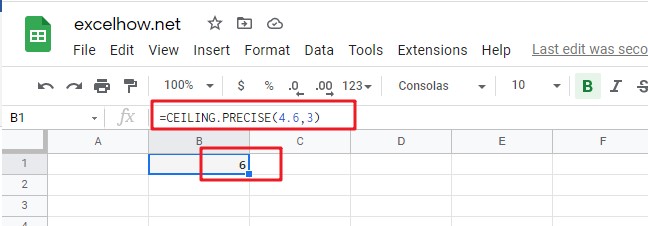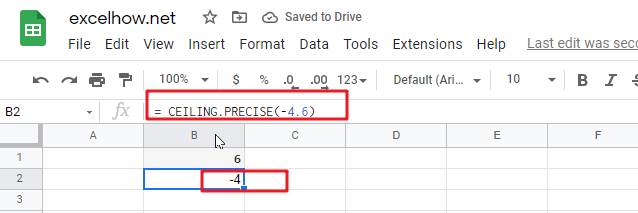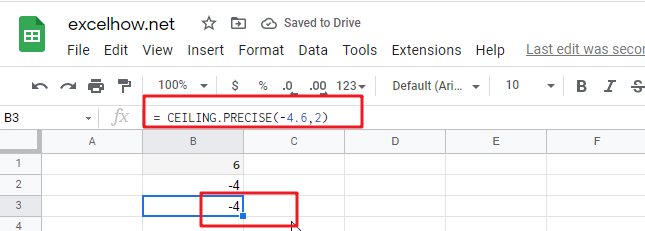# ExcelHow

This post will guide you how to use Google Sheets CEILING.PRECISE function with syntax and examples.

## Description

The Google Sheets CEILING.PRECISE function returns a given number rounded up to the nearest multiple of a given number of significance or returns a number that is rounded up to the nearest integer number.

The CEILING.PRECISE function is a build-in function in Google Sheets and it is categorized as a MATH function.

## Syntax

The syntax of the CEILING.PRECISE function is as below:

=CEILING.PRECISE (number, [significance])

Where the CEILING.PRECISE function arguments are:

• number – This is a required argument. The number that you want to round up.
• significance – This is an optional argument. The multiple of significance to which you want to round a number to. If the significance argument is omitted, the default value will be set to 1.

Note:

• If either number or significance arguments are non-numeric, the CEILING.PRECISE function will return the #VALUE! Error.
• Regardless of the sign of the number, the number is rounded up.
• If the number or the significance argument is zero, the CEILING.PRECISE function will return zero.

## Google Sheets CEILING.PRECISE Function Examples

The below examples will show you how to use google sheets CEILING.PRECISE Function to round a number up to nearest multiple.

1# to round 4.6 up to nearest multiple of 3, enter the following formula in Cell B1.

`=CEILING.PRECISE(4.6,3)`2# to round -4.6 up to nearest multiple of 1, enter the following formula in Cell B2.

`= CEILING.PRECISE(-4.6)`3# to round -4.6 up to nearest multiple of 2, enter the following formula in Cell B3.

`= CEILING.PRECISE(-4.6,2)`